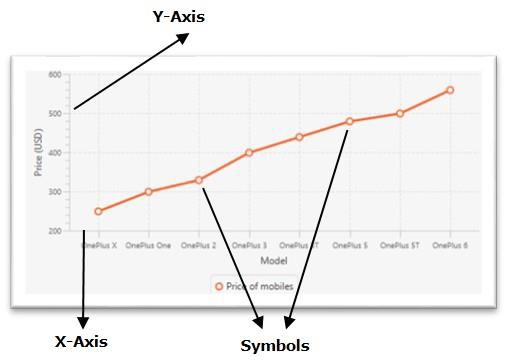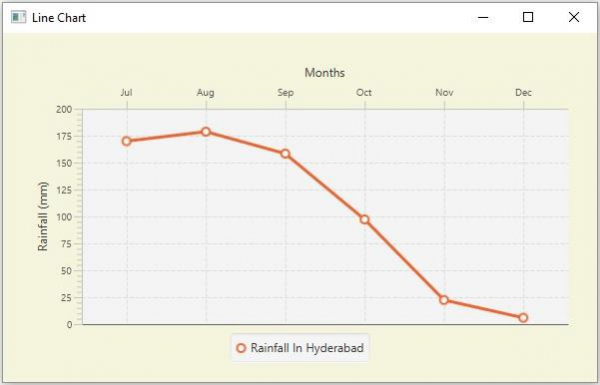# How to move the x-axis to top in a JavaFX line chart?

JavaFXObject Oriented ProgrammingProgramming

Inline chart, the data values have represented a series of points connected by a line. In JavaFX, you can create a line chart by instantiating the javafx.scene.chart.LineChart class.

By default,

• A JavaFX Line chart contains symbols pointing out the values in the series along the x-axis. Typically, these are small circles.

• The X-Axis on the bottom in the plot.

• Y-Axis on the left.## Moving the X-Axis to top

The Axis class (superclass of all axes) has a property named side, this specifies the side of the plot at which you need to have the current axis (left, right, top-bottom). You can set the value to this property using the setSide() method. This method accepts one of the following values as a parameter −

• Side.BOTTOM

• Side.TOP

• Side.LEFT

• Side.RIGHT

To move the X-Axis to top, invoke the setSide() method on the object of the XAxis of your plot by passing the value Side.TOP as a parameter.

## Example

import javafx.application.Application;
import javafx.geometry.Insets;
import javafx.geometry.Side;
import javafx.scene.Scene;
import javafx.stage.Stage;
import javafx.scene.chart.CategoryAxis;
import javafx.scene.chart.LineChart;
import javafx.scene.chart.NumberAxis;
import javafx.scene.chart.XYChart;
import javafx.scene.layout.StackPane;
public class LineChartAxisShift extends Application {
public void start(Stage stage) {
//Defining the x an y axes
CategoryAxis xAxis = new CategoryAxis();
NumberAxis yAxis = new NumberAxis();
//Setting labels for the axes
xAxis.setLabel("Months");
yAxis.setLabel("Rainfall (mm)");
//Creating a line chart
LineChart linechart = new LineChart(xAxis, yAxis);
//Preparing the data points for the line1
XYChart.Series series = new XYChart.Series();
//Setting the name to the line (series)
//Setting the data to Line chart
//Shifting the X-axis
xAxis.setSide(Side.TOP);
//Creating a stack pane to hold the chart
StackPane pane = new StackPane(linechart);
pane.setStyle("-fx-background-color: BEIGE");
//Setting the Scene
Scene scene = new Scene(pane, 595, 350);
stage.setTitle("Line Chart");
stage.setScene(scene);
stage.show();
}
public static void main(String args[]){
launch(args);
}
}

## Output